## Example Questions

### Example Question #8 : How To Add Odd Numbers

Solve: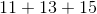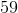Explanation: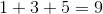Since there is no tens digit to carry over, proceed to add the tens digits: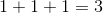The answer is.

### Example Question #9 : How To Add Odd Numbers

At a certain high school, everyone must take either Latin or Greek. There aremore students taking Latin than there are students taking Greek. If there are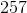students taking Greek, how many total students are there?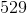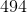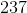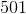Explanation:

If there arestudents taking Greek, then there are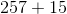or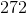students taking Latin. However, the question asks how many total students there are in the school, so you must add these two values together to get: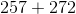ortotal students.

### Example Question #1 : Even / Odd Numbers

Find the sum of 13 and 19.Explanation:

Rewrite the question in a mathematical expression.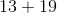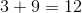Since this number is larger than, carry over thein tens digit when adding the next term.

Add the tens digit with the carry over.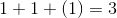Combine the tens digit and the ones digit. The answer is.

### Example Question #2 : Even / Odd Numbers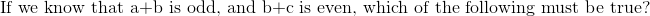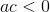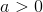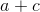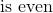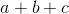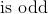Explanation:

In order to get an odd result from an addition, we must have one odd and one even number, thus you know from the first point about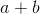that only one of the two values is odd. Now, to get an even result, you can have two evens or two odds. So, let's presume that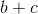has two odd values, this means thatmust be even. Thus, you have: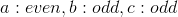Now, if we presume thathas two even values, we must then know thatis odd.  Thus, we have: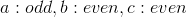First of all, you can eliminate the two answers that say that a given value is positive or negative. This cannot be told from our data. Next, it cannot be the case thatis even. It will always be odd (hence, the correct answer is this). Finally, it cannot be thatis even always. In the second case above, you will have two even numbers added together, given you an even. Then, you will add in an odd, giving you an odd.